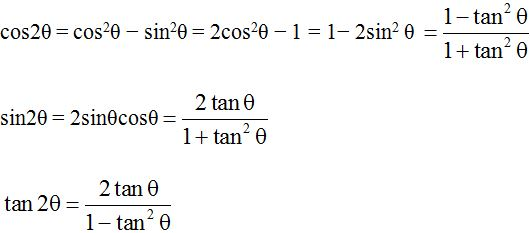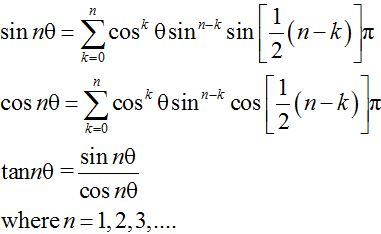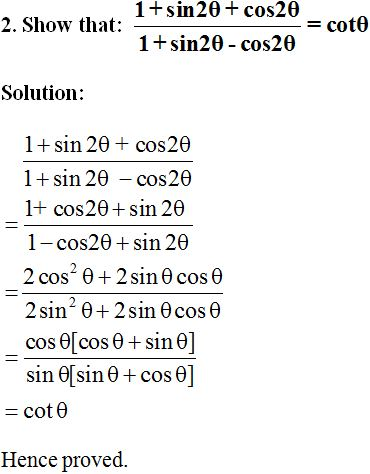# Angle Formulas: Definition, Formula, Questions & MoreCheck definition and formulas for central angle, double angle and multiple angles. Solved examples for more clarification of formulas are also provided here.

Angle: The measure of the opening between two intersecting lines is called an angle.

Unit: The unit of angle is radians or degrees.

Angle formula: There are different types of formulas for calculating the measurements of different angles. Let us discuss the major ones below:

Central Angle Formula: Central angle is the angle formed by two arms with the center of a circle as the vertex. The two arms form two radii of the circle intersecting the arc of the circle at different points.

The central angle formula is used to find the angle between two radii of a circle.

Central Angle Formula is represented as:

Double Angle Formulas:

Double angle formulas are named so because they involve trigonometric functions of double angles, i.e., sin2θ, cos2θ and tan2θ.

There are three main double angle formulas as below:where θ is the angle in a right-angled triangle.

Multiple Angle Formulas:

Multiple angles are of the form sinnθ, cosnθ, and tannθ. Sine, tangent and cosine are the general functions for the multiple angle formula.Some examples showing the application of angle formulas are given below:

1. Find the angle of a segment in a circle if the arc length is 7π and the radius is 9 units.

Solution:

Given, Arc length =  7π

Using Central Angles Formula,

Angle = (Arc Length × 360o)/2πr degrees

Angle = {(7π × 360o)/2π × 9} degrees

= 140 degrees.

Therefore, the angle of the segment is 140 degrees.To get more such useful articles and important resources for CBSE Board Exam or Competitive Exam preparations, visit jagranjosh.com.